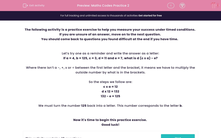# Maths Codes Practice 2

In this practice exercise, students will replace letters with numbers and solve problems using BIDMAS under timed conditions.This content is premium and exclusive to EdPlace subscribers.Key stage:  KS 2

Curriculum topic:   Exam-Style Questions: Verbal Reasoning

Curriculum subtopic:   Exam-Style Questions: Maths Codes

Difficulty level:#### Worksheet Overview

If you are unsure of an answer, move on to the next question.

You should come back to questions you found difficult at the end if you have time.

Let’s try one as a reminder and write the answer as a letter:

If a = 4, b = 125, c = 3, d = 11 and e = 7, what is d (c x a) - e?

Where there isn’t a -, +, x or ÷ between the first letter and the bracket, it means we have to multiply the outside number by what is in the brackets.

So the steps we follow are:

c x a = 12

d x 12 = 132

132 - e = 125

We must turn the number 125 back into a letter. This number corresponds to the letter b.

Now it's time to begin this practice exercise.

Good luck!

### What is EdPlace?

We're your National Curriculum aligned online education content provider helping each child succeed in English, maths and science from year 1 to GCSE. With an EdPlace account you’ll be able to track and measure progress, helping each child achieve their best. We build confidence and attainment by personalising each child’s learning at a level that suits them.

Get started